# Methods and formulas for Predict for Stability Study for random batches

Select the method or formula of your choice.

## Fitted value and SE fit for random batch

The fitted value is the predicted y or, which is the mean response value for the given predictor values using the estimated regression equation.

The standard error of the fitted values in the mixed model are the square roots of the diagonal elements of the matrix that follows:
where

### Notation

TermDescription
Zin x mi martix of known codings for the ith random effect in the model
Z'transpose of Z
yresponse data
Xdesign matrix, including the constant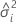variance component of the ith random factor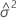variance component for error
Inidentity matrix with n rows and columns

## Prediction interval

The range in which the predicted response for a new observation is expected to fall. The calculation of the prediction interval depends on whether you compute the interval for the marginal fit or for the conditional fit.

### Marginal fit

where

The degrees of freedom for the t-statistic are given by this formula:

where

### Conditional fit

where

The degrees of freedom for the t-statistic are:

where

### Notation

TermDescription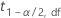1–α/2 quantile from the t distribution with the given degrees of freedom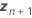vector of the new values of the random predictors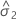variance component for error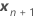vector of new values of the fixed predictorsvariance component of the ith random factor
Im identity matrix with m rows and columns
mnumber of columns in the design matrix to represent the ith random term in the model
c number of random effects in the model
Zin x mi design matrix for the ith random effect in the model
Z'itranspose of Zi

## Confidence interval

The range in which the estimated mean response for a given set of predictor values is expected to fall.

The standard error of the fitted values in the mixed model are the square roots of the diagonal elements of this matrix:

where

The degrees of freedom use this formula when batch is a random factor:

where

### Notation

TermDescription
t1-α/2, df1–α/2 quantile from the t distribution with the given degrees of freedom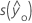standard error of the fitted value
Xdesign matrix, including the constant
X' transpose of Xvariance component for errorvariance component of the ith random factor
Zin x mi matrix of known codings for the ith random effect in the model
Zi' transpose of Zi
Inidentity matrix with n rows and columns
xi predictor values for the fit or prediction
W asymptotic variance-covariance matrix of the variance component for error
cnumber of random effects in the model
By using this site you agree to the use of cookies for analytics and personalized content.  Read our policy